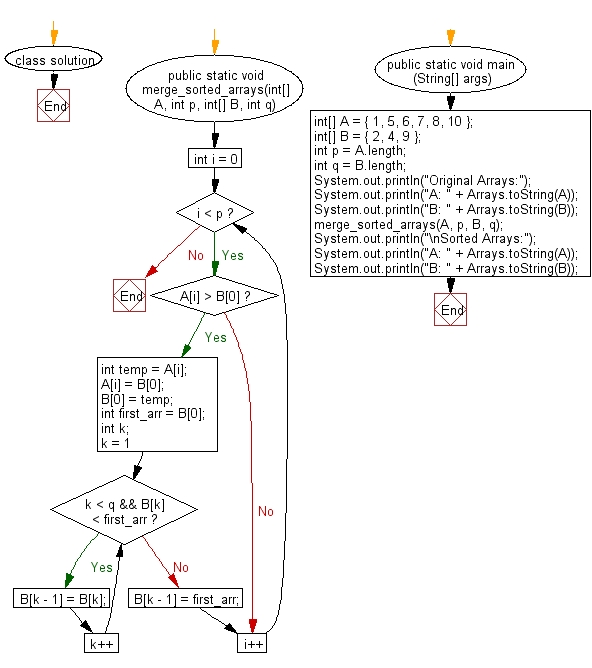﻿ Java: Merge elements of A with B by maintaining the order# Java Array Exercises: Merge elements of A with B by maintaining the sorted order

## Java Array: Exercise-58 with Solution

Given two sorted arrays A and B of size p and q, write a Java program to merge elements of A with B by maintaining the sorted order i.e. fill A with first p smallest elements and fill B with remaining elements.

Example:
Input :
int[] A = { 1, 5, 6, 7, 8, 10 }
int[] B = { 2, 4, 9 }
Output:
Sorted Arrays:
A: [1, 2, 4, 5, 6, 7]
B: [8, 9, 10]

Sample Solution:

Java Code:

``````import java.util.Arrays;

class solution
{
public static void merge_sorted_arrays(int[] A, int p, int[] B, int q)
{

for (int i = 0; i < p; i++)
{
if (A[i] > B)
{
int temp = A[i];
A[i] = B;
B = temp;

int first_arr = B;
int k;
for (k = 1; k < q && B[k] < first_arr; k++) {
B[k - 1] = B[k];
}

B[k - 1] = first_arr;
}
}
}

public static void main (String[] args)
{
int[] A = { 1, 5, 6, 7, 8, 10 };
int[] B = { 2, 4, 9 };
int p = A.length;
int q = B.length;

System.out.println("Original Arrays:");
System.out.println("A: " + Arrays.toString(A));
System.out.println("B: " + Arrays.toString(B));

merge_sorted_arrays(A, p, B, q);

System.out.println("\nSorted Arrays:");
System.out.println("A: " + Arrays.toString(A));
System.out.println("B: " + Arrays.toString(B));
}
}
```
```

Sample Output:

```Original Arrays:
A: [1, 5, 6, 7, 8, 10]
B: [2, 4, 9]

Sorted Arrays:
A: [1, 2, 4, 5, 6, 7]
B: [8, 9, 10]
```

Flowchart:Java Code Editor:

Improve this sample solution and post your code through Disqus

What is the difficulty level of this exercise?

Test your Programming skills with w3resource's quiz.

﻿

## Java: Tips of the Day

countOccurrences

Counts the occurrences of a value in an array.

Use Arrays.stream().filter().count() to count total number of values that equals the specified value.

```public static long countOccurrences(int[] numbers, int value) {
return Arrays.stream(numbers)
.filter(number -> number == value)
.count();
}
```

Ref: https://bit.ly/3kCAgLb